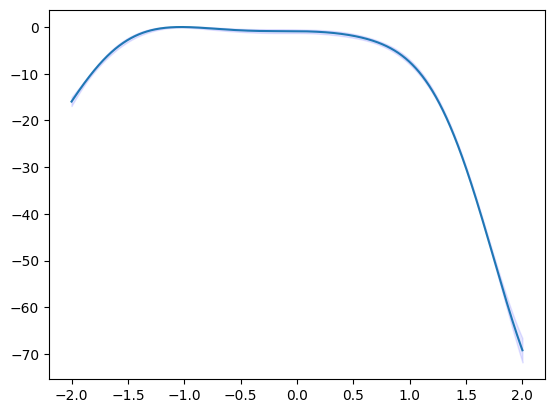# Basic usage of PHYSBO

## Introduction

In this tutorial, we will introduce how to define the simulator class and find the minimum value of a one-dimensional function using PHYSBO.

First, we will import PHYSBO.

:

import physbo


## Defining the search space

In the following example, the search space X is defined as a grid chopped by window_num=10001 divisions from x_min = -2.0 to x_max = 2.0. Note that X must be in window_num x d ndarray format (d is the number of dimensions, in this case one). In this case, d is the number of dimensions, in this case two, so we use reshape to transform it.

:

#In
import numpy as np
import scipy
import physbo
import itertools

#In
#Create candidate
window_num=10001
x_max = 2.0
x_min = -2.0

X = np.linspace(x_min,x_max,window_num).reshape(window_num, 1)


## Defining the simulator class

Here, we define the simulator class to set as the objective function.

In this case, the problem is to find the minimum $$x$$ such that $$f(x) = 3 x^4 + 4 x ^3 + 1.0$$ (the answer is $$x=-1.0$$).

In the simulator class, we define the __call__ function (or __init__ if there are initial variables, etc.). (If there are initial variables, define __init__.) The action indicates the index number of the grid to be retrieved from the search space, and is generally in the form of an ndarray so that multiple candidates can be calculated at once. In this case, we choose one candidate point from X as action_idx=action to calculate only one candidate at a time. Since PHYSBO is designed to find the maximum value of the objective function, it returns the value of f(x) at the candidate point multiplied by -1.

:

# Declare the class for calling the simulator.
class simulator:

def __call__(self, action ):
action_idx = action
x = X[action_idx]
fx = 3.0*x**4 + 4.0*x**3 + 1.0
fx_list.append(fx)
x_list.append(X[action_idx])

print ("*********************")
print ("Present optimum interactions")

print ("x_opt=", x_list[np.argmin(np.array(fx_list))])

return -fx


## Performing optimization

### Setting policy

First, set the optimization policy.

Next, set test_X to the matrix of search candidates (numpy.array).

:

# set policy
policy = physbo.search.discrete.policy(test_X=X)

# set seed
policy.set_seed(0)


When policy is set, no optimization is done yet. Execute the following methods on policy to optimize it.

• random_search.

• bayes_search.

If you specify the simulator and the number of search steps in these methods, the following loop will be executed for the number of search steps.

1. Select the next parameter to be executed from the candidate parameters.

2. Execute simulator with the selected parameters.

The default number of parameter returned by i) is one, but it is possible to return multiple parameters in one step. See the section “Searching for multiple candidates at once” for details.

Also, instead of running the above loop inside PHYSBO, it is possible to control i) and ii) separately from the outside. In other words, it is possible to propose the next parameter to be executed from PHYSBO, evaluate its objective function value in some way outside PHYSBO (e.g., by experiment rather than numerical calculation), propose it in some way outside PHYSBO, and register the evaluated value in PHYSBO. For more details, please refer to the “Running Interactively” section of the tutorial.

### Bayesian Optimization

Next, we run the Bayesian optimization as follows.

argument.

• max_num_probes: Number of search steps.

• simulator: The simulator of the objective function (an object of class simulator).

• score: The type of acquisition function. You can specify one of the following

• TS (Thompson Sampling)

• EI (Expected Improvement)

• PI (Probability of Improvement)

• interval: The hyperparameters are trained at the specified interval. If a negative value is specified, no hyperparameter will be learned. 0 means that hyperparameter learning will be performed only in the first step.

• num_rand_basis: Number of basis functions. 0 means that a normal Gaussian process without Bayesian linear model will be used.

[ ]:

res = policy.bayes_search(max_num_probes=50, simulator=simulator(), score='TS',
interval=0, num_rand_basis=500)


## Checking the results

The search result res is returned as an object of the history class (physbo.search.discrete.results.history).
The following is a reference to the search results.
• res.fx : The history of evaluated values of simulator (objective function).

• res.chosen_actions: The history of action IDs (parameters) when the simulator was evaluated.

• fbest, best_action= res.export_all_sequence_best_fx(): The history of best values and their action IDs (parameters) for all timings when the simulator was evaluated.

• res.total_num_search: Total number of simulator evaluations.

Let’s plot the objective function value and the best value at each step.
res.fx and best_fx should range up to res.total_num_search, respectively.
:

import matplotlib.pyplot as plt
%matplotlib inline

:

plt.plot(res.fx[0:res.total_num_search])

:

[<matplotlib.lines.Line2D at 0x7f816129d820>]:

best_fx, best_action = res.export_all_sequence_best_fx()
plt.plot(best_fx)

:

[<matplotlib.lines.Line2D at 0x7f8130b30f70>]## Serializing the results

The search results can be saved to an external file using the save method.

:

res.save('search_result.npz')

:

del res


Load the saved result file as follows:

:

res = physbo.search.discrete.results.history()


Finally, the candidate with the best score can be displayed as follows. You can see that we have arrived at the correct solution $$x=-1$$.

:

print(X[int(best_action[-1])])

[-1.002]


## Prediction

policy serves get_post_fmean and get_post_fcov methods for calculating mean value and variance of Gaussian process (post distribution).

:

mean = policy.get_post_fmean(X)
var = policy.get_post_fcov(X)
std = np.sqrt(var)
xs = X[:,0]

ax = plt.subplot()
ax.plot(xs, mean)
ax.fill_between(xs, mean-std, mean+std, color="blue", alpha=.1)

:

<matplotlib.collections.PolyCollection at 0x7f81613d8370>## Acquisition function

policy serves get_score method for calculating acquisition function.

:

scores = policy.get_score(mode="EI", xs=X)
plt.plot(scores)

:

[<matplotlib.lines.Line2D at 0x7f8130b84070>]## Parallelization

PHYSBO can calculate acquisition functions for candidates in parallel by using MPI via mpi4py . To enable MPI parallelization, pass a MPI communicator such as MPI.COMM_WORLD to a keyword argument, comm of the constructor of the policy.

:

# from mpi4py import MPI
# policy = physbo.search.discrete.policy(test_X=X, comm=MPI.COMM_WORLD)